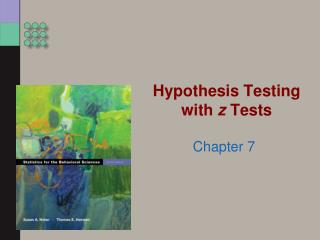DownloadDownload PresentationHypothesis Testing with z Tests

# Hypothesis Testing with z Tests

Télécharger la présentation## Hypothesis Testing with z Tests

- - - - - - - - - - - - - - - - - - - - - - - - - - - E N D - - - - - - - - - - - - - - - - - - - - - - - - - - -
##### Presentation Transcript

1. Hypothesis Testing with z Tests Chapter 7

2. The z Table • Benefits of standardization: allowing fair comparisons • z table: provides percentage of scores between the mean and a given z score

3. Raw Scores, z Scores, and Percentages Step 1: Convert raw score to z score Step 2: Look up area in Table The table presents area between the Mean and z and beyond the mean and z.

4. From Percentages to z Scores • Step 1: Use the z table in reverse, taking a percentage and converting it into a z score. • Step 2: Convert the z score to a raw score using the formula.

5. Sketching the Normal Curve • The benefits of sketching the normal curve: • Stays clear in memory; minimizes errors • Practical reference • Condenses the information

6. The Standardized z Distribution

7. Calculating the Percentile for a Positive z Score

8. Calculating the Percentage Above a Positive z Score

9. Calculating the Percentile for a Negative z Score

10. Calculating the Percentage Above a Negative z Score

11. Calculating a Score from a Percentile

12. Check Your Learning • If the population mean is 10 and the standard deviation is 2: • What is the percentile rank of a sample mean of 6? of 11? • What percentage of the samples would score higher than a score of 6? of 11?

13. The Assumptions and the Steps of Hypothesis Testing • Requirements to conduct analyses • Assumption: characteristic about a population that we are sampling necessary for accurate inferences

14. Parametric v. Nonparametric Tests • Parametric tests: inferential statistical test based on assumptions about a population • Nonparametric tests: inferential statistical test not based on assumptions about the population

15. An Example of the z Test • The z test • When we know the population mean and the standard deviation • The z test • The six steps of hypothesis testing • H0, H1 • One-tailed vs. two-tailed tests

16. Determining Critical Values for a z Distribution – One tailed or two-tailed test for significance?

17. Making a Decision

18. Check Your Learning • IQ scores are designed to have a mean of 100 and a standard deviation of 15. • Assume the class mean is 114. • Go through the six steps of hypothesis testing.

19. “Dirty” Data • “Dirty” Data: Missing data, misleading data, and outliers • Misleading data: The famous butterfly ballot used in Florida during the 2000 presidential election showed the of the arrangement of items on a page.

20. Cleaning “Dirty” Data • Judgment calls need to be made. • The best solution is to report everything so that other researchers can assess the trade-offs. • The best way to address the problem of dirty data is replication.